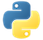# PartitionPydoc

Separates elements in a collection into multiple output collections. The partitioning function contains the logic that determines how to separate the elements of the input collection into each resulting partition output collection.

The number of partitions must be determined at graph construction time. You cannot determine the number of partitions in mid-pipeline

## Examples

In the following examples, we create a pipeline with a `PCollection` of produce with their icon, name, and duration. Then, we apply `Partition` in multiple ways to split the `PCollection` into multiple `PCollections`.

`Partition` accepts a function that receives the number of partitions, and returns the index of the desired partition for the element. The number of partitions passed must be a positive integer, and it must return an integer in the range `0` to `num_partitions-1`.

### Example 1: Partition with a function

In the following example, we have a known list of durations. We partition the `PCollection` into one `PCollection` for every duration type.

``````import apache_beam as beam

durations = ['annual', 'biennial', 'perennial']

def by_duration(plant, num_partitions):
return durations.index(plant['duration'])

with beam.Pipeline() as pipeline:
annuals, biennials, perennials = (
pipeline
| 'Gardening plants' >> beam.Create([
{'icon': '🍓', 'name': 'Strawberry', 'duration': 'perennial'},
{'icon': '🥕', 'name': 'Carrot', 'duration': 'biennial'},
{'icon': '🍆', 'name': 'Eggplant', 'duration': 'perennial'},
{'icon': '🍅', 'name': 'Tomato', 'duration': 'annual'},
{'icon': '🥔', 'name': 'Potato', 'duration': 'perennial'},
])
| 'Partition' >> beam.Partition(by_duration, len(durations))
)

annuals | 'Annuals' >> beam.Map(lambda x: print('annual: {}'.format(x)))
biennials | 'Biennials' >> beam.Map(
lambda x: print('biennial: {}'.format(x)))
perennials | 'Perennials' >> beam.Map(
lambda x: print('perennial: {}'.format(x)))``````

Output:

``````perennial: {'icon': '🍓', 'name': 'Strawberry', 'duration': 'perennial'}
biennial: {'icon': '🥕', 'name': 'Carrot', 'duration': 'biennial'}
perennial: {'icon': '🍆', 'name': 'Eggplant', 'duration': 'perennial'}
annual: {'icon': '🍅', 'name': 'Tomato', 'duration': 'annual'}
perennial: {'icon': '🥔', 'name': 'Potato', 'duration': 'perennial'}``````Run code nowView source code

### Example 2: Partition with a lambda function

We can also use lambda functions to simplify Example 1.

``````import apache_beam as beam

durations = ['annual', 'biennial', 'perennial']

with beam.Pipeline() as pipeline:
annuals, biennials, perennials = (
pipeline
| 'Gardening plants' >> beam.Create([
{'icon': '🍓', 'name': 'Strawberry', 'duration': 'perennial'},
{'icon': '🥕', 'name': 'Carrot', 'duration': 'biennial'},
{'icon': '🍆', 'name': 'Eggplant', 'duration': 'perennial'},
{'icon': '🍅', 'name': 'Tomato', 'duration': 'annual'},
{'icon': '🥔', 'name': 'Potato', 'duration': 'perennial'},
])
| 'Partition' >> beam.Partition(
lambda plant, num_partitions: durations.index(plant['duration']),
len(durations),
)
)

annuals | 'Annuals' >> beam.Map(lambda x: print('annual: {}'.format(x)))
biennials | 'Biennials' >> beam.Map(
lambda x: print('biennial: {}'.format(x)))
perennials | 'Perennials' >> beam.Map(
lambda x: print('perennial: {}'.format(x)))``````

Output:

``````perennial: {'icon': '🍓', 'name': 'Strawberry', 'duration': 'perennial'}
biennial: {'icon': '🥕', 'name': 'Carrot', 'duration': 'biennial'}
perennial: {'icon': '🍆', 'name': 'Eggplant', 'duration': 'perennial'}
annual: {'icon': '🍅', 'name': 'Tomato', 'duration': 'annual'}
perennial: {'icon': '🥔', 'name': 'Potato', 'duration': 'perennial'}``````Run code nowView source code

### Example 3: Partition with multiple arguments

You can pass functions with multiple arguments to `Partition`. They are passed as additional positional arguments or keyword arguments to the function.

In machine learning, it is a common task to split data into training and a testing datasets. Typically, 80% of the data is used for training a model and 20% is used for testing.

In this example, we split a `PCollection` dataset into training and testing datasets. We define `split_dataset`, which takes the `plant` element, `num_partitions`, and an additional argument `ratio`. The `ratio` is a list of numbers which represents the ratio of how many items will go into each partition. `num_partitions` is used by `Partitions` as a positional argument, while `plant` and `ratio` are passed to `split_dataset`.

If we want an 80%/20% split, we can specify a ratio of `[8, 2]`, which means that for every 10 elements, 8 go into the first partition and 2 go into the second. In order to determine which partition to send each element, we have different buckets. For our case `[8, 2]` has 10 buckets, where the first 8 buckets represent the first partition and the last 2 buckets represent the second partition.

First, we check that the ratio list’s length corresponds to the `num_partitions` we pass. We then get a bucket index for each element, in the range from 0 to 9 (`num_buckets-1`). We could do `hash(element) % len(ratio)`, but instead we sum all the ASCII characters of the JSON representation to make it deterministic. Finally, we loop through all the elements in the ratio and have a running total to identify the partition index to which that bucket corresponds.

This `split_dataset` function is generic enough to support any number of partitions by any ratio. You might want to adapt the bucket assignment to use a more appropriate or randomized hash for your dataset.

``````import apache_beam as beam
import json

def split_dataset(plant, num_partitions, ratio):
assert num_partitions == len(ratio)
bucket = sum(map(ord, json.dumps(plant))) % sum(ratio)
total = 0
for i, part in enumerate(ratio):
total += part
if bucket < total:
return i
return len(ratio) - 1

with beam.Pipeline() as pipeline:
train_dataset, test_dataset = (
pipeline
| 'Gardening plants' >> beam.Create([
{'icon': '🍓', 'name': 'Strawberry', 'duration': 'perennial'},
{'icon': '🥕', 'name': 'Carrot', 'duration': 'biennial'},
{'icon': '🍆', 'name': 'Eggplant', 'duration': 'perennial'},
{'icon': '🍅', 'name': 'Tomato', 'duration': 'annual'},
{'icon': '🥔', 'name': 'Potato', 'duration': 'perennial'},
])
| 'Partition' >> beam.Partition(split_dataset, 2, ratio=[8, 2])
)

train_dataset | 'Train' >> beam.Map(lambda x: print('train: {}'.format(x)))
test_dataset | 'Test' >> beam.Map(lambda x: print('test: {}'.format(x)))``````

Output:

``````train: {'icon': '🍓', 'name': 'Strawberry', 'duration': 'perennial'}
train: {'icon': '🥕', 'name': 'Carrot', 'duration': 'biennial'}
test: {'icon': '🍆', 'name': 'Eggplant', 'duration': 'perennial'}
test: {'icon': '🍅', 'name': 'Tomato', 'duration': 'annual'}
train: {'icon': '🥔', 'name': 'Potato', 'duration': 'perennial'}``````Run code nowView source code

• Filter is useful if the function is just deciding whether to output an element or not.
• ParDo is the most general elementwise mapping operation, and includes other abilities such as multiple output collections and side-inputs.
• CoGroupByKey performs a per-key equijoin.Pydoc